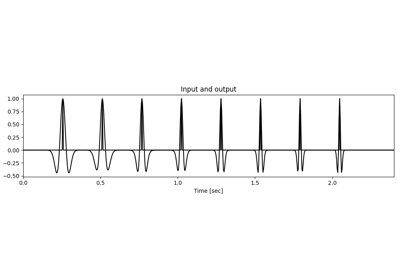# pylops.signalprocessing.NonStationaryConvolve1D#

class pylops.signalprocessing.NonStationaryConvolve1D(dims, hs, ih, axis=-1, dtype='float64', name='C')[source]#

1D non-stationary convolution operator.

Apply non-stationary one-dimensional convolution. A varying compact filter is provided on a coarser grid and on-the-fly interpolation is applied in forward and adjoint modes.

Parameters
dims

Number of samples for each dimension

hsnumpy.ndarray

Bank of 1d compact filters of size $$n_\text{filts} \times n_h$$. Filters must have odd number of samples and are assumed to be centered in the middle of the filter support.

ihtuple

Indices of the locations of the filters hs in the model (and data). Note that the filters must be regularly sampled, i.e. $$dh=\text{diff}(ih)=\text{const.}$$

axisint, optional

Axis along which convolution is applied

dtypestr, optional

Type of elements in input array.

namestr, optional

Name of operator (to be used by pylops.utils.describe.describe)

Raises
ValueError

If filters hs have even size

ValueError

If ih is not regularly sampled

ValueError

If ih is outside the bounds defined by dims[axis]

Notes

The NonStationaryConvolve1D operator applies non-stationary one-dimensional convolution between the input signal $$d(t)$$ and a bank of compact filter kernels $$h(t; t_i)$$. Assuming an input signal composed of $$N=5$$ samples, and filters at locations $$t_1$$ and $$t_3$$, the forward operator can be represented as follows:

$\begin{split}\mathbf{y} = \begin{bmatrix} \hat{h}_{0,0} & h_{1,0} & \hat{h}_{2,0} & h_{3,0} & \hat{h}_{4,0} \\ \hat{h}_{0,1} & h_{1,1} & \hat{h}_{2,1} & h_{3,1} & \hat{h}_{4,1} \\ \vdots & \vdots & \vdots & \vdots & \vdots \\ \hat{h}_{0,4} & h_{1,4} & \hat{h}_{2,4} & h_{3,4} & \hat{h}_{4,4} \\ \end{bmatrix} \begin{bmatrix} x_0 \\ x_1 \\ \vdots \\ x_4 \end{bmatrix}\end{split}$

where $$\mathbf{h}_1 = [h_{1,0}, h_{1,1}, \ldots, h_{1,N}]$$ and $$\mathbf{h}_3 = [h_{3,0}, h_{3,1}, \ldots, h_{3,N}]$$ are the provided filter, $$\hat{\mathbf{h}}_0 = \mathbf{h}_1$$ and $$\hat{\mathbf{h}}_4 = \mathbf{h}_3$$ are the filters outside the range of the provided filters (which are extrapolated to be the same as the nearest provided filter) and $$\hat{\mathbf{h}}_2 = 0.5 \mathbf{h}_1 + 0.5 \mathbf{h}_3$$ is the filter within the range of the provided filters (which is linearly interpolated from the two nearest provided filter on either side of its location).

In forward mode, each filter is weighted by the corresponding input and spread over the output. In adjoint mode, the corresponding filter is element-wise multiplied with the input, all values are summed together and put in the output.

Attributes
shapetuple

Operator shape

explicitbool

Operator contains a matrix that can be solved explicitly (True) or not (False)

Methods

 __init__(dims, hs, ih[, axis, dtype, name]) adjoint() apply_columns(cols) Apply subset of columns of operator cond([uselobpcg]) Condition number of linear operator. conj() Complex conjugate operator div(y[, niter, densesolver]) Solve the linear problem $$\mathbf{y}=\mathbf{A}\mathbf{x}$$. dot(x) Matrix-matrix or matrix-vector multiplication. eigs([neigs, symmetric, niter, uselobpcg]) Most significant eigenvalues of linear operator. matmat(X) Matrix-matrix multiplication. matvec(x) Matrix-vector multiplication. reset_count() Reset counters rmatmat(X) Matrix-matrix multiplication. rmatvec(x) Adjoint matrix-vector multiplication. todense() Return dense matrix. toimag([forw, adj]) Imag operator toreal([forw, adj]) Real operator tosparse() Return sparse matrix. trace([neval, method, backend]) Trace of linear operator. transpose()

## Examples using pylops.signalprocessing.NonStationaryConvolve1D#Non-stationary Convolution

Non-stationary Convolution